If you find any mistakes, please make a comment! Thank you.

## Definition of the Heisenberg group over a field

Solution to Abstract Algebra by Dummit & Foote 3rd edition Chapter 1.4 Exercise 1.4.11

Let $F$ be a field, and define the Heisenberg group $H(F)$ over, $F$ by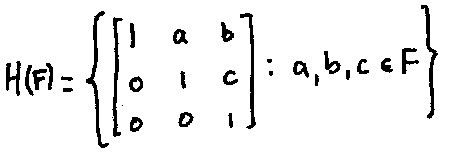(1) Show that $H(F)$ is closed under matrix multiplication. Demonstrate explicitly that $H(F)$ is always non-abelian.
(2) Given $X \in H(F)$, find an explicit formula for $X^{-1}$ and deduce that $H(F)$ is closed under inversion.
(3) Prove the associative law for $H(F)$ under matrix multiplication. Deduce that $H(F)$ is a group.
(4) Find the order of each element of the finite group $H(\mathbb{F}_2)$.
(5) Prove that every nonidentity element of the group $H(\mathbb{R})$ has infinite order.

Solution:

(1) Let $X, Y \in H(F)$, with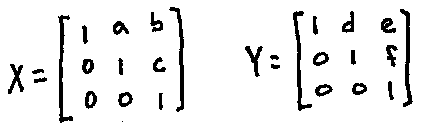Note that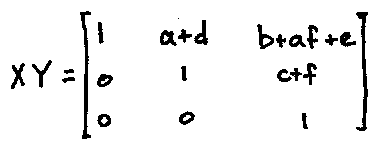so that $XY \in H(F)$. Thus $H(F)$ is closed under matrix multiplication. Now since $F$ is a field, we always have $0,1 \in F$. Then we have $A, B \in H(F)$, where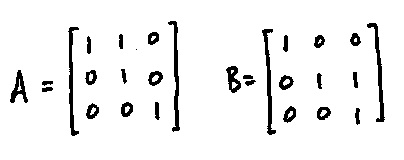But note that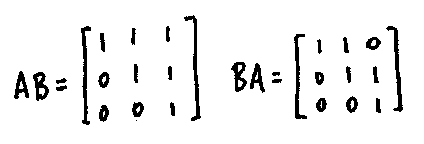So that $H(F)$ is nonabelian for all $F$ (provided $0 \neq 1$).

(2) We have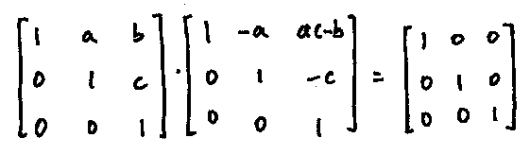so that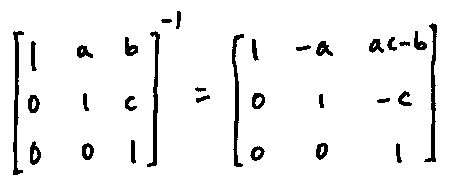Hence $H(F)$ is closed under matrix inversion.

(3) Note that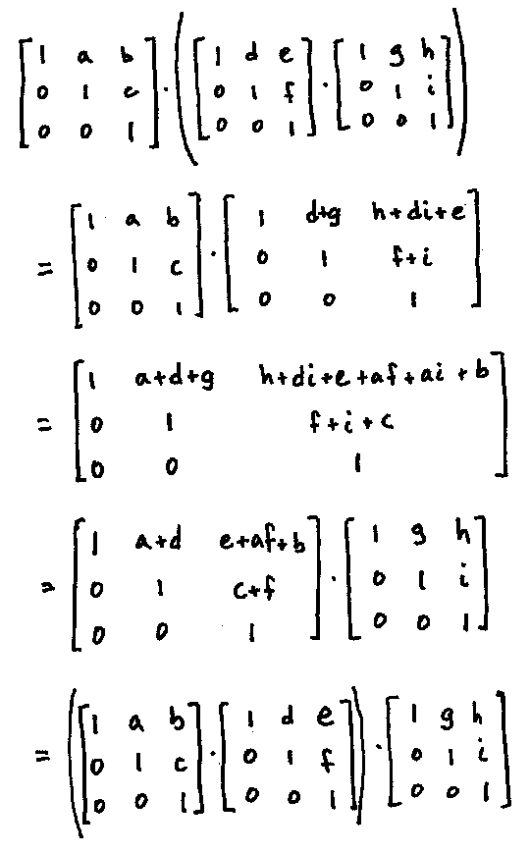so that matrix multiplication is associative on $H(F)$. Thus $H(F)$ is a group under matrix multiplication; moreover, it is clear that $|H(F)| = |F|^3$.

(4) We will denote the elements of $H(\mathbb{F}_2) by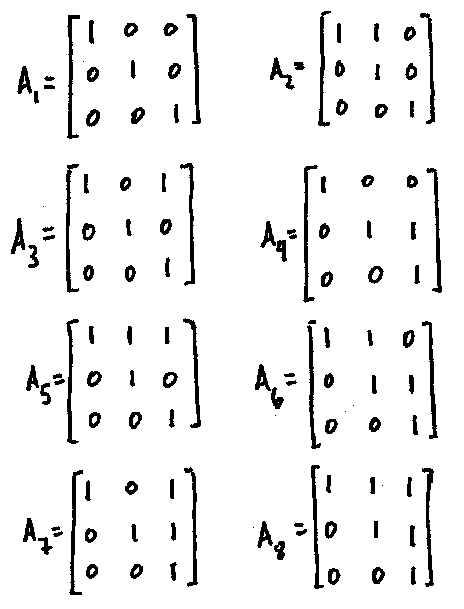Now$A_2^2 = A_3^2 = A_4^2 = A_5^2 = A_7^2 = I$, so these elements have order 2. Since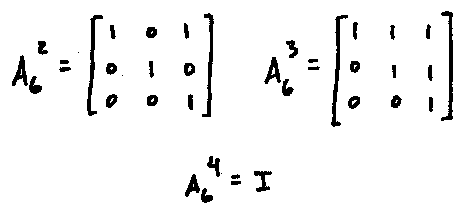we have that$|A_6| = 4$, and likewise since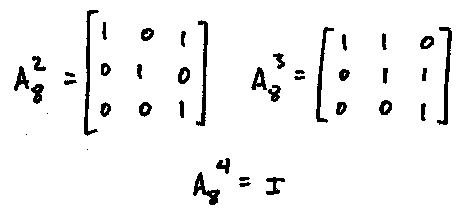we have$|A_8| = 4$. (5) We claim that for all$a,b,c \in \mathbb{R}$and integers$n \geq 1$,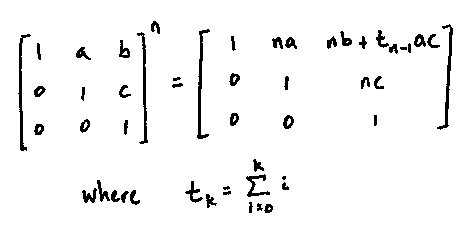which we prove by induction on$n$. For the base case, we have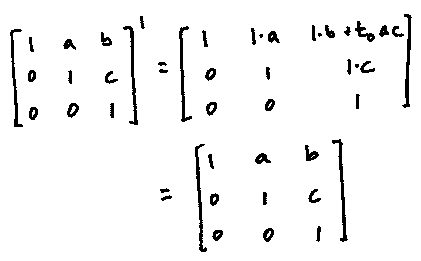Now suppose the statement holds for$n$. Then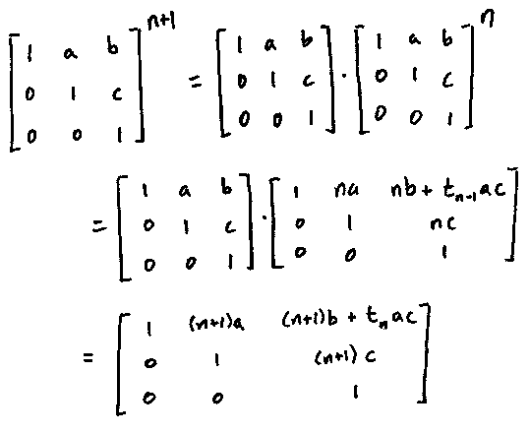so that it holds also for$n+1$. Thus the statement is proved. Now consider an arbitrary nonidentity element$X \in H(\mathbb{R})$, where$X$is defined as above. If either$a$or$c$is nonzero, then the (1,2) or the (2,3) element of$X^n$is nonzero for all positive$n$. If$a = c = 0$, then we have$b \neq 0$and so the (1,3) entry of$X^n$is nonzero for all positive$n\$.